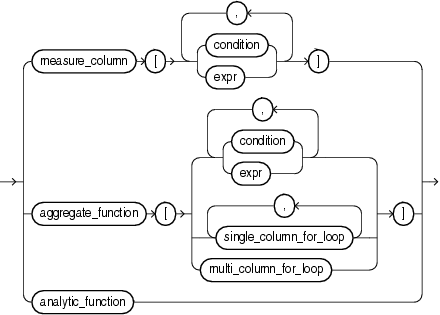## 5.12 Model Expressions

A model expression is used only in the `model_clause` of a `SELECT` statement and then only on the right-hand side of a model rule. It yields a value for a cell in a measure column previously defined in the `model_clause`. For additional information, refer to model_clause.

model_expression::=Description of the illustration model_expression.eps

When you specify a measure column in a model expression, any conditions and expressions you specify must resolve to single values.

When you specify an aggregate function in a model expression, the argument to the function is a measure column that has been previously defined in the `model_clause`. An aggregate function can be used only on the right-hand side of a model rule.

Specifying an analytic function on the right-hand side of the model rule lets you express complex calculations directly in the `model_clause`. The following restrictions apply when using an analytic function in a model expression:

• Analytic functions can be used only in an `UPDATE` rule.

• You cannot specify an analytic function on the right-hand side of the model rule if the left-hand side of the rule contains a `FOR` loop or an `ORDER` `BY` clause.

• The arguments in the `OVER` clause of the analytic function cannot contain an aggregate.

• The arguments before the `OVER` clause of the analytic function cannot contain a cell reference.

See Also:

The MODEL clause: Examples for an example of using an analytic function on the right-hand side of a model rule

When `expr` is itself a model expression, it is referred to as a nested cell reference. The following restrictions apply to nested cell references:

• Only one level of nesting is allowed.

• A nested cell reference must be a single-cell reference.

• When `AUTOMATIC` `ORDER` is specified in the `model_rules_clause`, a nested cell reference can be used on the left-hand side of a model rule only if the measures used in the nested cell reference remain static.

The model expressions shown below are based on the `model_clause` of the following `SELECT` statement:

```SELECT country,prod,year,s
FROM sales_view_ref
MODEL
PARTITION BY (country)
DIMENSION BY (prod, year)
MEASURES (sale s)
IGNORE NAV
UNIQUE DIMENSION
RULES UPSERT SEQUENTIAL ORDER
(
s[prod='Mouse Pad', year=2000] =
s['Mouse Pad', 1998] + s['Mouse Pad', 1999],
s['Standard Mouse', 2001] = s['Standard Mouse', 2000]
)
ORDER BY country, prod, year;
```

The following model expression represents a single cell reference using symbolic notation. It represents the sales of the Mouse Pad for the year 2000.

```s[prod='Mouse Pad',year=2000]
```

The following model expression represents a multiple cell reference using positional notation, using the `CV` function. It represents the sales of the current value of the dimension column `prod` for the year 2001.

```s[CV(prod), 2001]
```

The following model expression represents an aggregate function. It represents the sum of sales of the Mouse Pad for the years between the current value of the dimension column `year` less two and the current value of the dimension column `year` less one.

`SUM(s)['Mouse Pad',year BETWEEN CV()-2 AND CV()-1]`

See Also: